### Want a basic technical understanding of solar PV project terminology? Read on…

If you’re an experienced installer, none of this information will be new to you. If you’re new to solar, it will be helpful. But keep in mind, we’ll be skimming the surface.

We’re going to begin with the basic terms. This is very important for design because you need to understand the concepts before you start applying real numbers to a design. It will also help with sales because it will help you explain some basic concepts to curious customers.

Performing high-quality and efficient site visits is absolutely critical to the success of profitable solar projects, especially residential projects. You need to be able to capture all of the information you need to quote the system correctly, design the project, and tell the installation crew what to expect. An efficient site visit process will lead to smooth operations and profitable jobs while a complex or disorganized process can lead to unprofitable jobs and a lot of confusion.

Power

Power is an AMOUNT of energy. It’s the measurement of energy, measured in kilowatts (kW). Power is measured in an instant. Most of the sizing done in solar PV design (conductors, inverters, fuses, etc.) is based on how much power will be passing through a specific component of the system. Because power is measured in an instant, it can vary widely over time and from minute to minute.

Power (watts) = current (Amps) X voltage (volts)

Energy

Energy is the is the actual work done by power. It is measure in kilowatt-hours (kWh). Consumers pay for kWh. It’s a measure of power over time.

Power (kW) X Time (hours) = Energy (kWh)

Current

Electricity is the flow of negatively charged electrons. The current is the amount of negatively charged electrons in a specific part of a circuit.

Many people find it useful to use a water analogy when discussing electrical terms. In the water example, it’s useful to think of a dam with a pipe at the bottom where water can flow out.  The amount of water that can pass through a slice of the pipe, in other words the area of the cross-section of the pipe, is analogous to electric current.

Voltage

Voltage is a measure of the force or pressure of the electric current in a circuit. It’s measured in volts.  Electrons of the same material WANT to be homogeneous, i.e. they want to be evenly spread out. Thus, if one area has less electrons then another, the electrons will move in an attempt to equalize. This flow is what created a voltage potential and causes electrons to move.

To use the water example again, if the size of the pipe at the bottom of a dam is a measure of current, the height of the dam is a measure of voltage. Higher water behind the dam creates more pressure.

Resistance

Electrical resistance is the resistance of the flow of electricity through a conductor. It does not reduce the current flow of electrons (how many electrons there are in the circuit), but it does reduce the voltage (how fast they’re going). It is measured in ohms.

Voltage Drop (volts) = Current (amps) X Resistance (ohms)

Series Circuit

A series circuit is when one negative and positive of each power source or appliance are connected together.

Remember, CURRENT is constant and Voltage ADDS in series circuits.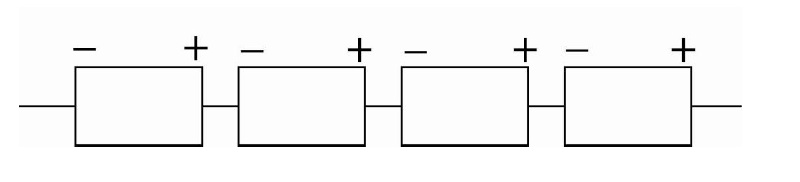Parallel Circuit

In a parallel circuit, all of the positives are connected together and the negative are connected together, each separately.

In parallel circuits, CURRENT ADDS and voltage stays constant.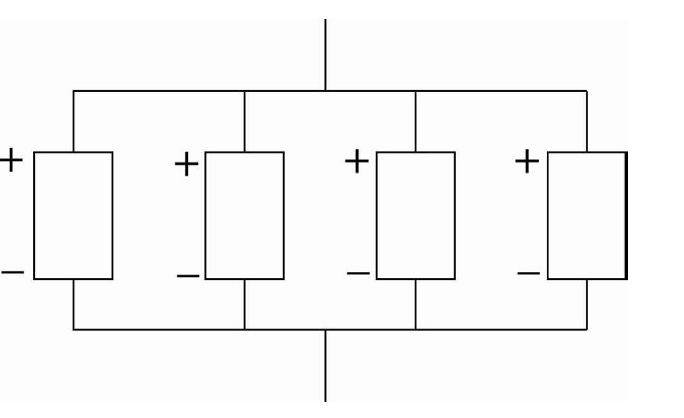AC Current

AC refers to alternating current. It refers to electrical systems where the voltage and current are constantly changing between positive and negative. A complete “cycle” is completed when when the current reaches returns to either the peak or trough of the wave. Frequency is measured in hertz (Hz) and is measured in number of cycles per second. The power in the US is operated at 60 Hz.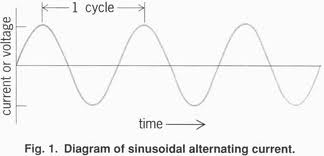DC Current

DC stands for direct current. DC is the type of electricity where the voltage and current stay constant over time. Typical DC applications are batteries, solar modules, and wind turbines.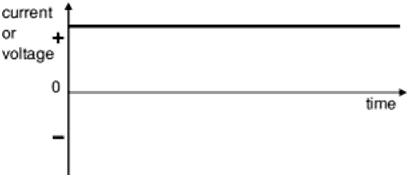Calculating and Correcting Solar Resource

Irradiance is the amount of solar radiation falling on a particular area at any given time. It is a RATE. It’s a measure of POWER, in that it’s an instantaneous term that does not consider time. Remember the difference between power and energy.

It is measured in watts per square meter.

Irradiation is a measure of solar energy, the amount of irradiance that falls on a location over time.

Irradiation is measured in kWh / square meter / day.

Solar Energy in the US

The below pictures shows that amount of solar irradiation that falls on the various surfaces across the US depending on average local weather circumstances.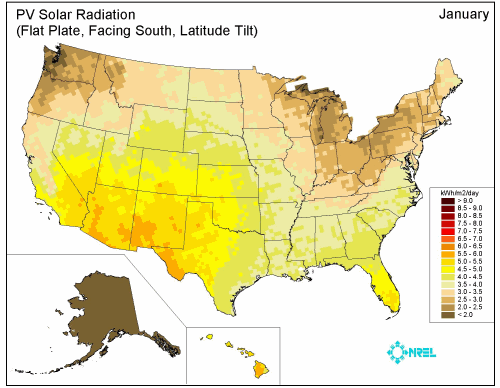Horizontal Tilt

The tilt angle from the sun is the angle from the horizon to the sun. Solar PV modules will produce the most energy when the sun is shining directly onto them, from a 90-degree angle. Thus, all else equal, for fixed PV modules the best tilt angle will be the same as the latitude of the site. For example, if the PV site is at 44 N, the best tilt will be 44 degrees. However, most roofs and and commercial racking are not at 44 degrees, so you must apply correction factors for projects that are not at perfect tilts. We will discuss this in a later article.

Azimuth

The azimuth is the number of degrees from true south that the sun, or another object, is facing. It’s used when designing a solar PV system because due south will provide the best production, all else equal, over the course of a year. We’re not going to get into tracking systems in this series, so all of our arrays will be fixed. However, if the object is not directly south, you will need to apply correction factors that we will get to in later articles.

Magnetic Declination

Keep in mind that if you’re doing site visits with a magnetic compass you will need to correct your magnetic readings to find true south. The process is simple.

Determine your declination by looking at a diagram like the one below and finding your location.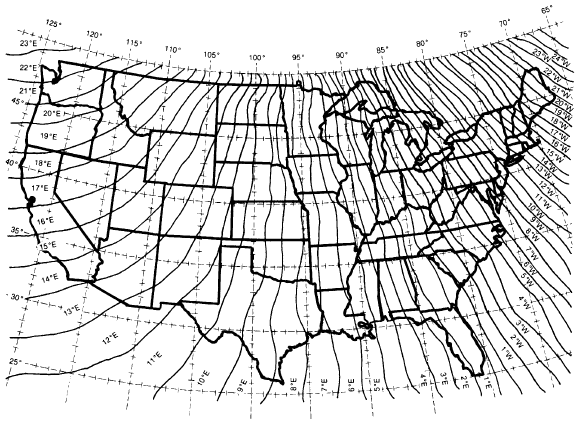EAST – Subtract. If your compass reading was 190 degrees and you lived in San Francisco, about 17 degrees east, you would need to subtract 17 degrees to find true south. You’re TRUE SOUTH reading would be 173 degrees.

WEST – Add. If you lived in Belfast, Maine (about 19 degrees west) and your compass reading was 165 degrees, you would need to ADD 19 degrees to get a TRUE SOUTH of 184 degrees.

Solar Module Terms: You will need to understand the terms below when sizing your system.

Voc: Volts open circuit is the maximum voltage a solar module can ever make when it has no load on it. Voc is used when sizing solar arrays along with temperature coefficients to determine worst-case voltage scenarios.

Vmp: Volts maximum power is the reading of the maximum volts a module can produce when under load under standing testing condition, STC, irradiance levels (1000 W / M2) . If you look at the curve below, the Vmp would be somewhere in curve on the right in the bend. It will be on the place in the curve the creates the most power (volts times amps). The number is actually rather to difficult to calculate exactly and can change rapidly from second to second as the current changes.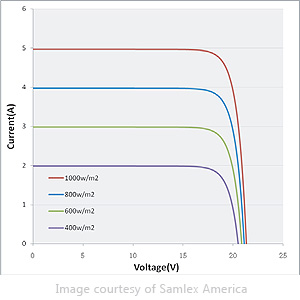Isc: Amps short circuits it the maximum amount of amps that a solar module could produce. You will find Isc on the X axis of the  graph above where there is no voltage and thus no power being produced.

Imp: Amps max power, like volts max power, is the current point on the power curve when the module is producing maximum power.

You’ll find the material above on the back of every individual solar PV module and it is standard information that manufacturers and distributors will tell you about their product. Below is a product description for two Sharp modules from AEE Solar. All the data is public and available on AEE’s website.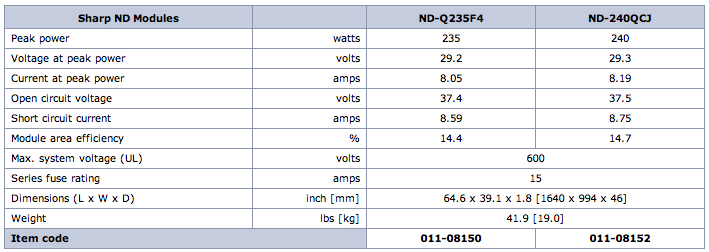Temperature and Voltage: It’s important to understand the relationship between temperature and voltage in solar modules for design purposes. While temperature does have a slight impact on current, it’s considered to be negligible. However, temperature has a large impact on voltage. When you are determining the maximum number of solar modules in a string, based on the inverter’s acceptable voltage window, you will need to take into account expected lowest temperature ranges that can increase voltage.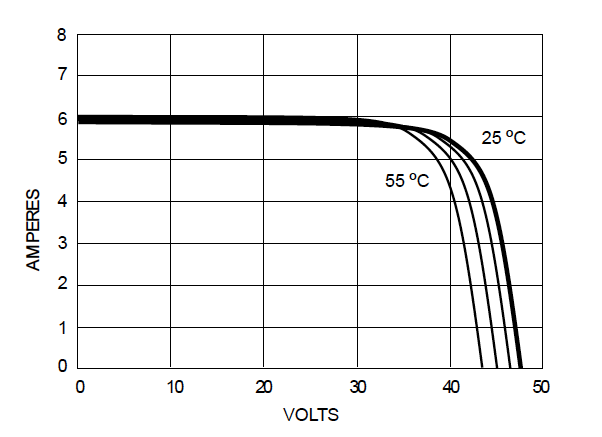Irradiance and Current: Irradiance and current also have a direct relationship. The amount of irradiance falling on a solar PV module will directly impact the current that module is producing.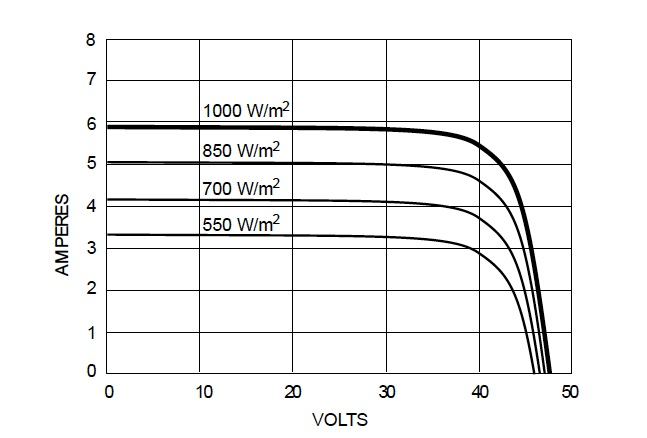To get more free information on solar sales and finance that is useful if you’re looking to start a solar business, check out our solar startup guide.